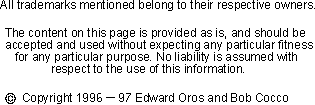Design Your Own Half Wave Vertical Antenna

The half wave antenna consists of a vertical radiator which is fed at the base of the antenna. A matching device of some sort must be added between the antenna and the feedline if you wish to feed it with coax. Adding a coil in series with the antenna at the base is one such method of matching.

So why would anyone use a halfwave antenna if they have to go through all that extra work? After all, a ground plane antenna provides a nicer match. There are a couple of answers. The first is GAIN. The computer shows a ground plane antenna (mounted 1 foot above ground) has a gain of 2.41 dBd lower than a dipole's gain (free space). The half wave antenna (mounted 1 foot above ground) shows only 1.56 dBd lower than a dipole (free space), so you've increase your overall gain .85 dB over the ground plane antenna!

After looking at those numbers you might be asking yourself, 'wait a minute, how can the half wave vertical have less gain than a dipole which is virtually the same antenna?' Well actually both antennas do have about the same gain. Gain is a relative thing, in the above paragraph, I'm comparing free space gain against above ground gain, two different things entirely. If you were to mount a dipole vertically 1 foot above ground too, you'd find that that its gain is 1.90 dB lower than the free space gain of the same antenna. The dipole and the half wave antenna do have the same gain (within .34 dB in this example).

The second reason you may want to use the half wave vertical is to obtain a lower angle of radiation. A ground plane mounted at 1 foot above ground has a radiation peak at 25 degrees, the half wave antenna radiation peak angle drops down to 20 degrees, making it a better dx antenna.

All antenna lengths depend on various factors. Some of these factors are:the height above ground, the diameter of the wire, nearby structures, the effects of other antennas in the area and even the conductivity of the soil.

This page allows you to calculate the length for a half wave antenna. It uses the standard formula, 468/f MHz (142.6464/f MHz for metric) to calculate the element lengths (This is the same as that of a dipole). If you've experimented with half wave antennas before and know of a better formula for your QTH, feel free to change the formula to suit. This formula is for a wire antenna. Of course if you construct your antenna out of tubing, the total length of the antenna will be shorter.
Enter the formula for the antenna calculation

Switch to:

Divided by Freq MHz

Your half wave antenna's vertical length is

Back to the Antenna Elmer